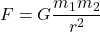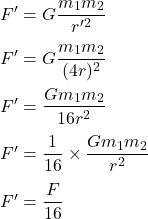## If the distance between the center of two objects is quadrupled. The gravitational force between the two objects will change by a fact

Question

If the distance between the center of two objects is quadrupled. The gravitational
force between the two objects will change by a factor of:
1) 16
2) 0.25
3) 4
4) 0.0625

in progress 0
6 months 2021-07-24T19:16:44+00:00 1 Answers 3 views 0

## Answers ( )

F’ = F/16

Explanation:

The gravitational force between masses is given by :If the distance between the center of two objects is quadrupled, r’ = 4r

New force will be :So, the new force will change by a factor of 16.